# Test: Digital Electronics Circuits- 2

## 25 Questions MCQ Test Basic Electronics Engineering for SSC JE (Technical) | Test: Digital Electronics Circuits- 2

Description
Attempt Test: Digital Electronics Circuits- 2 | 25 questions in 50 minutes | Mock test for Electrical Engineering (EE) preparation | Free important questions MCQ to study Basic Electronics Engineering for SSC JE (Technical) for Electrical Engineering (EE) Exam | Download free PDF with solutions
QUESTION: 1

Solution:
QUESTION: 2

Solution:
QUESTION: 3

### The characteristic equation of the T-flip-flop is given by

Solution:
QUESTION: 4

The initial contents of the 4 dit serial in-parallelout, right Shift, register shown in the given figureis 0110. After three clock pulses are applied, the contents of the shift register will be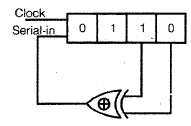Solution:
QUESTION: 5

With 4 Boolean variables, how many Boolean expressions can be formed?

Solution:
QUESTION: 6

A number is expressed in binary two's complement a 10011, Its decimal equivalent value is

Solution:
QUESTION: 7

The Boolean expression (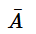+ B)(A+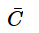)(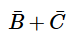) Simplifies to

Solution:
QUESTION: 8

The minimum number of NAND gates required to imnplement the Boolean function

A+ A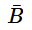+ AC is equal to

Solution:
QUESTION: 9

The addition of two binary variables A and B results into a SUM And a CARRY output.Consider the following expressions for the SUM and CARRY outputs.

1. SUM = A.B +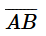2. SUM = A.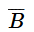+ A.3. CARRY = A.B

4. Carry = A +B

Which of these expressions are correct?

Solution:
QUESTION: 10

For a binary half subtractor having two inputs A and B, the correct sets of logical expressions for the output sets of logical expressions for the output D (= A minus B) and X (= borrow) are

Solution:
QUESTION: 11

Minimum number of J-K flip-flip- flops needed to construct a BCD counter is

Solution:
QUESTION: 12

The characteristic equation for the next state (Qn +1) of a J-K flip- flop is

Solution:
QUESTION: 13

The initial contents of the 4-bit series in- parallelout, right shift, shift register as shown in figure below are 0110. After 3 clock pulses the contents of the shift register will be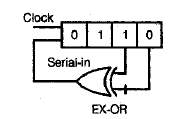Solution:
QUESTION: 14

How many 1's are present in the binary representation of (4 ×4096) + (9 × 256) + (7×16)+5 ?

Solution:
QUESTION: 15

Which IC is used for the implementation of 1-to-16 DEMUX?

Solution:

IC 74154 is used for the implementation of 1-to-16 DEMUX, whose output is inverted input.

QUESTION: 16

Which one of the following statements is correct?

Solution:
QUESTION: 17

Match List-I (Type of N-bit ADC) with List-II (Characteristics) and select the correct answer using the codes given below the lists: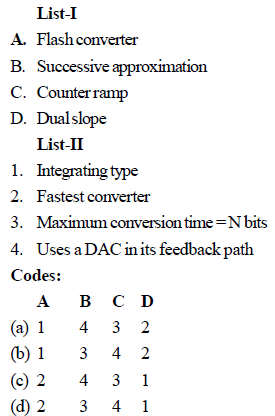Solution:
QUESTION: 18

If (2.3) base 4 +(1.2) base4 = (Y)base 4 ; what is the value of Y?

Solution:
QUESTION: 19

Given (135)base x +(144) base x = (323) base x

Solution:
QUESTION: 20

What is the output f(X,y) of the multiplexer resulting from the input logical values?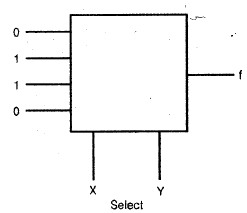Solution:
QUESTION: 21

Given below are three types of converters:

1. Successive approximation type

2. Weighted resistor type

Which of these types are D to A converters?

Solution:
QUESTION: 22

What is the Gray code word for the binary binary 101011?

Solution:
QUESTION: 23

If A and B are Boolean Variables, then what is (A+ B). (A+) equal to ?

Solution:
QUESTION: 24

What is the Boolean expression A Å B equivalent to?

Solution:
QUESTION: 25

A 1 ms pulse can be converted into a 10 ms pulse by using which one of the following?

Solution:Use Code STAYHOME200 and get INR 200 additional OFF Use Coupon Code